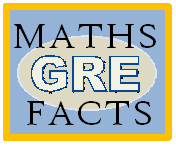## Did You Know these Facts about GRE Math?

~ By our Quantitative Reasoning FacultyApril is almost over and the countdown to the exam has already begun. You want a good overall score and if you’re an engineer, you are most probably thinking that getting 165 on Quant shouldn’t be too much of a problem (the typical engineer approaches maths questions with a raw “Just bring ‘em on” kind of arrogance and usually gets most questions right). But here’s the problem: sometimes even those with a strong background in maths may not cross the 160 mark – and when that happens, dreams of a score in the 325+ range come crashing down. To prevent that unhappy outcome, here are some basic insights about the way the math works on GRE.

One fundamental reason why some students don’t get the scores they should, is that they simply don’t understand the way the exam ‘TALKS’ maths. What this means is that the GRE test has its own way of defining mathematical terms. If you don’t understand the definitions used in the GRE exam, then time and time again you are going to end up making errors on questions you should have got right – and you are likely to end up feeling frustrated and demoralized. So, let’s have a look at a few basic differences between Indian maths and American math.

1. The First Surprise – GRE Numbers are neither Natural nor Whole!

In school (for us in India), the basic classification of numbers is this:

• Natural Numbers – {1, 2, 3, 4… ∞}
• Whole Numbers – {0, 1, 2, 3…∞}
• Integers – {-∞…,-1, 0, 1…∞}
• Fractions – p/q, q≠0, p, q e integers

But ETS never uses the term ‘Natural Numbers.’ They refer to these numbers as ‘Positive Integers’. Also, you won’t find the term ‘Whole Numbers,’ instead, ETS uses the term ‘Non-Negative Integers’. What you need to catch here is that in Quant, the negation of a negative is not always positive. It is either positive or ZERO!

2. Some More Surprises – Oddly Enough with Even Numbers, and Even with Odd Ones

Let’s have a look at a few other definitions that we learnt in school (and went by for years):

• Even Numbers – {2, 4, 6, 8…} in short, numbers which are divisible by 2
• Odd Numbers – {1, 3, 5, 7…} in short, numbers which are not divisible by 2

But when it comes to GRE, the definitions have a twist! According to ETS:

• Even Numbers – {…-8,-6, -4, -2, 0, 2, 4, 6, 8…}
• Odd Numbers – {…-7,-5, -3, -1, 1, 3, 5, 7…}

That means in the GRE exam, if the question says “x is an even number”, then you cannot really assume that ‘x’ has a positive value. In fact, you should remember that ‘x’ can be either positive or ZERO or NEGATIVE! The same rule applies to odd numbers as well.

3. One More Thing to Factor in – Along with Multiple Problems

Similarly, if you were asked to list the factors of 10, your answer would be {1, 2, 5 and 10}, which would be fine in an Indian exam. In the GRE, however, this would be regarded as an incomplete (and therefore wrong) answer. The reason is that the ETS expects you to consider the negative factors as well! Hence, in the GRE the correct statement of the factors of 10 would be:{1, 2, 5, 10,-1,-2,-5 and -10}.

The same is true when listing multiples as well, i.e., if you are asked to enumerate the multiples of 5, your answer set should contain {…-15,-10,-5,0,5,10,15…} and not {5,10,15,20…} only!

So, now are you ready for this?

Now that you have the necessary ‘inside information,’ you should be! So, try solving this question:

How many positive integers, less than 20, are either an even multiple of 2 or, a multiple of 9 or, the sum of a positive multiple of 2 and a positive multiple of 9?

Let’s see how many integers you can come up with! (see answer here)